# Graphing whole number functions Online Quiz

Following quiz provides Multiple Choice Questions (MCQs) related to Graphing whole number functions. You will have to read all the given answers and click over the correct answer. If you are not sure about the answer then you can check the answer using Show Answer button. You can use Next Quiz button to check new set of questions in the quiz.### Explanation

Step 1:

From the graph plot, we get the coordinates of the points as (0, 0), (1, 6), (2, 9), (3, 10), and (4, 4)

Step 2:

Making a table of the x and y values, we get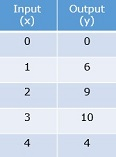### Explanation

Step 1:

From the table, we get the coordinates of the points as (0, 2), (1, 7), (2, 12), (3, 17), and (4, 22)

Step 2:

Making a plot of these points, we get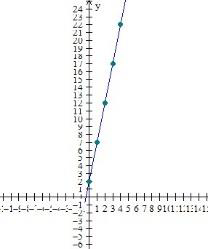### Explanation

Step 1:

From the table, we get the coordinates of the points as (0, 0), (2, 4), (4, 8), (6, 12), and (8, 16)

Step 2:

Making a plot of these points, we get### Explanation

Step 1:

From the graph plot, we get the coordinates of the points as (0, 0), (1, 5), (2, 9), (3, 7), and (4, 10)

Step 2:

Making a table of the x and y values, we get### Explanation

Step 1:

From the graph plot, we get the coordinates of the points as (0, 3), (1, 5), (2, 8), (3, 9), and (4, 12)

Step 2:

Making a table of the x and y values, we get### Explanation

Step 1:

From the table, we get the coordinates of the points as (0, 1), (1, 4), (2, 7), (3, 10), and (4, 13)

Step 2:

Making a plot of these points, we get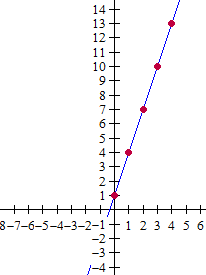### Explanation

Step 1:

From the graph plot, we get the coordinates of the points as (0, 0), (1, 4), (2, 9), (3, 5), and (4, 10)

Step 2:

Making a table of the x and y values, we get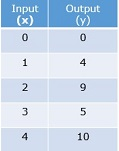### Explanation

Step 1:

From the table, we get the coordinates of the points as (0, 0), (1, 0.5), (3, 1.5), (5, 2.5), and (7, 3.5)

Step 2:

Making a plot of these points, we get### Explanation

Step 1:

From the table, we get the coordinates of the points as (0, 3), (2, 5), (3, 6), (5, 8), and (8, 11)

Step 2:

Making a plot of these points, we get### Explanation

Step 1:

From the graph plot, we get the coordinates of the points as (0, 0), (1, 8), (2, 1), (3, 10), and (4, 2)

Step 2:

Making a table of the x and y values, we get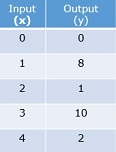graphing_whole_number_functions.htm